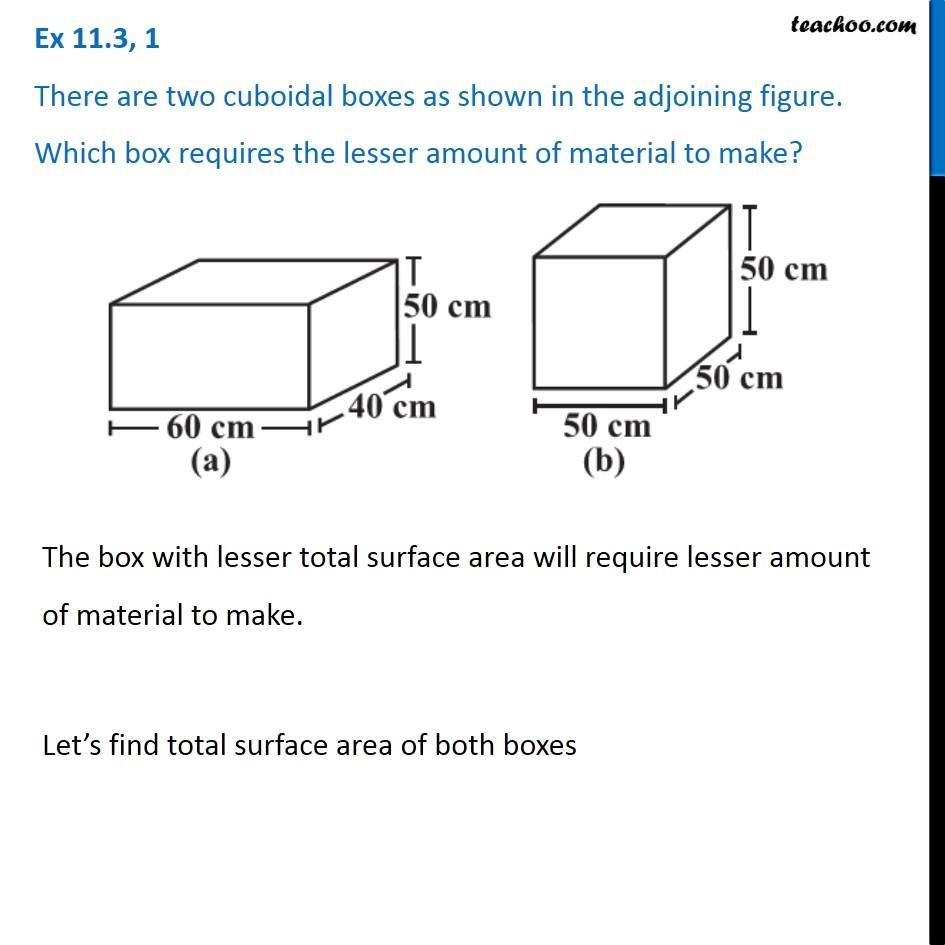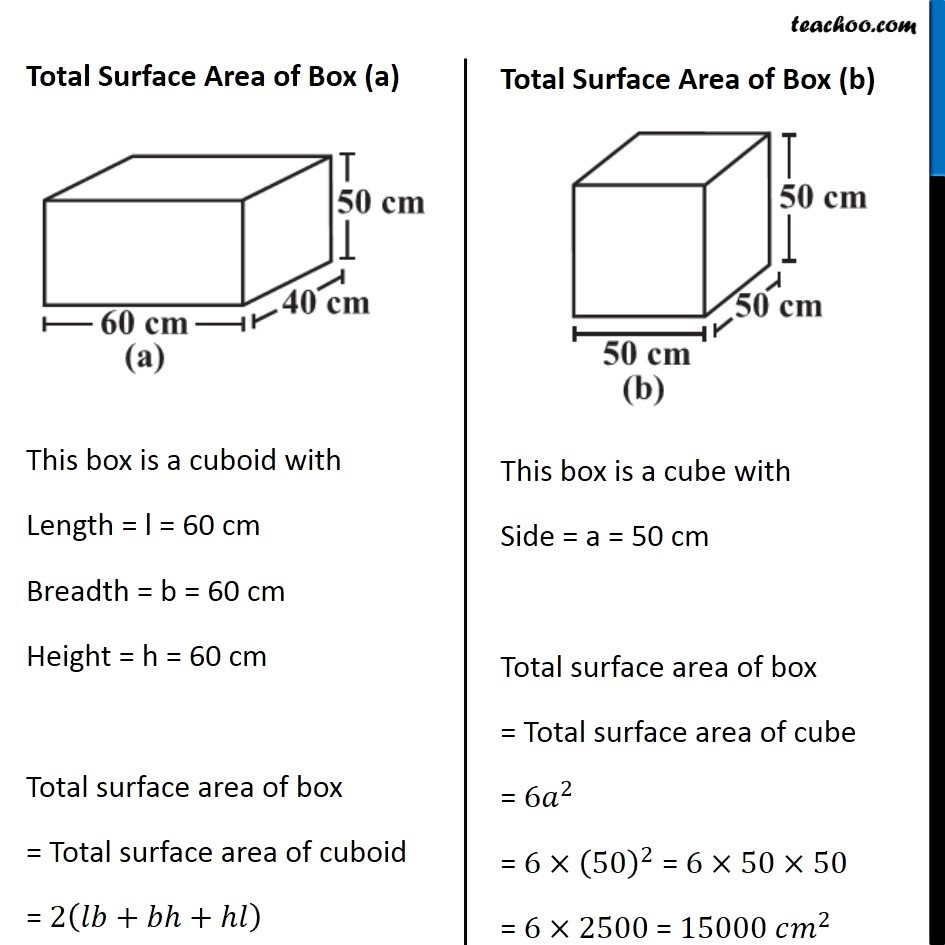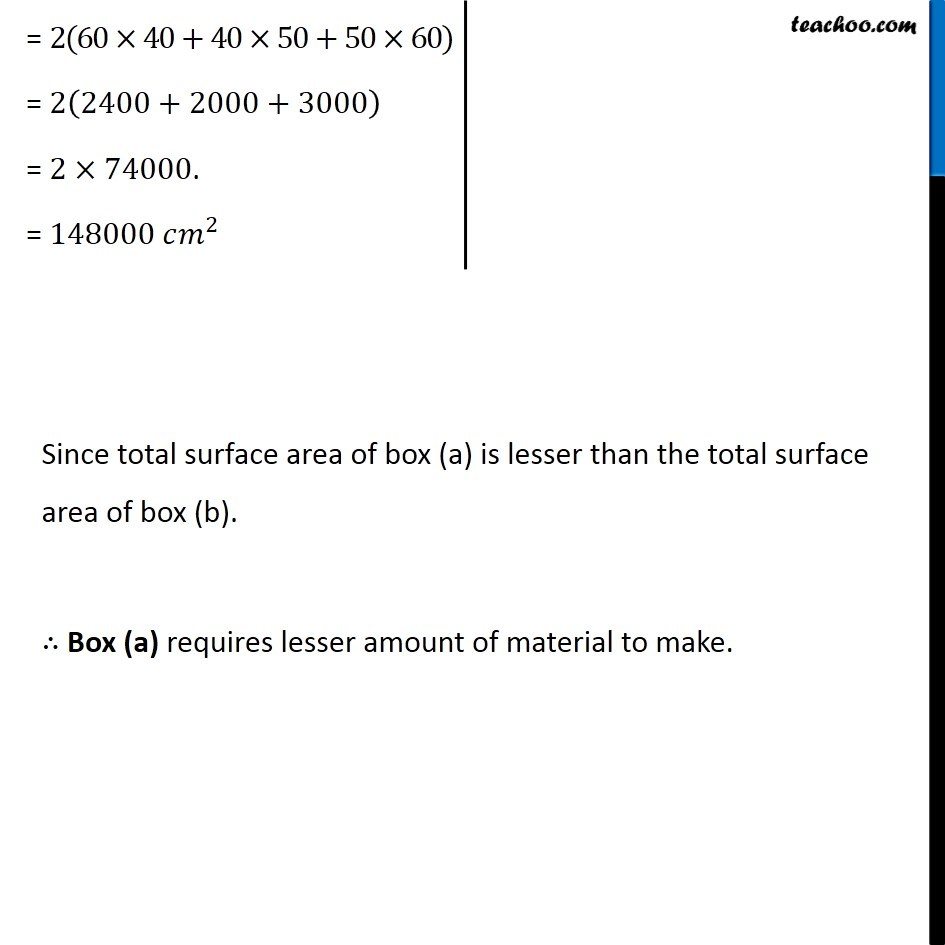Surface Area of Cuboid

Chapter 11 Class 8 Mensuration
Concept wiseIntroducing your new favourite teacher - Teachoo Black, at only ₹83 per month

### Transcript

Ex 11.3, 1 There are two cuboidal boxes as shown in the adjoining figure. Which box requires the lesser amount of material to make? The box with lesser total surface area will require lesser amount of material to make. Let’s find total surface area of both boxes Total Surface Area of Box (a) This box is a cuboid with Length = l = 60 cm Breadth = b = 60 cm Height = h = 60 cm Total surface area of box = Total surface area of cuboid = 2(𝑙𝑏+𝑏ℎ+ℎ𝑙) Total Surface Area of Box (b) This box is a cube with Side = a = 50 cm Total surface area of box = Total surface area of cube = 6𝑎^2 = 6×(50)^2 = 6×50×50 = 6×2500 = 15000 𝑐𝑚^2 = 2(60×40+40×50+50×60) = 2(2400+2000+3000) = 2×74000. = 148000 𝑐𝑚^2 Since total surface area of box (a) is lesser than the total surface area of box (b). ∴ Box (a) requires lesser amount of material to make.